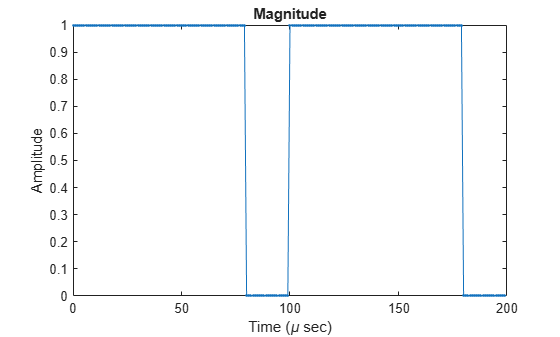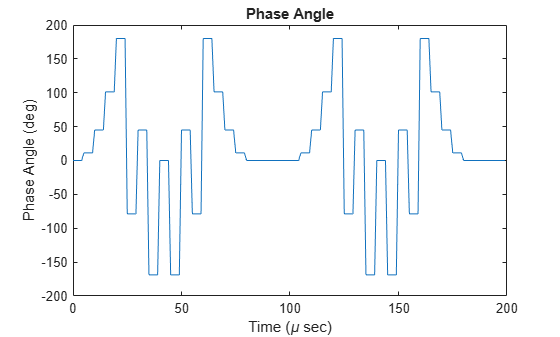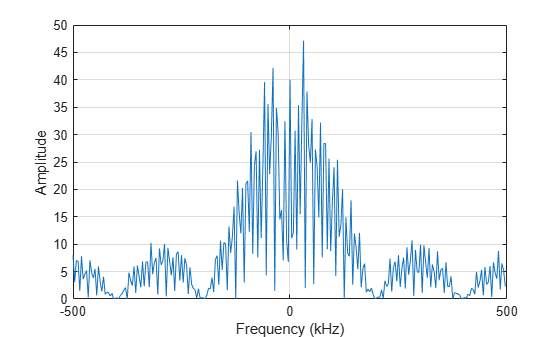Documentation

# phased.PhaseCodedWaveform

Phase-coded pulse waveform

## Description

The PhaseCodedWaveform object creates a phase-coded pulse waveform.

To obtain waveform samples:

1. Define and set up your phase-coded pulse waveform. See Construction.

2. Call step to generate the phase-coded pulse waveform samples according to the properties of phased.PhaseCodedWaveform. The behavior of step is specific to each object in the toolbox.

### Note

Starting in R2016b, instead of using the step method to perform the operation defined by the System object™, you can call the object with arguments, as if it were a function. For example, y = step(obj,x) and y = obj(x) perform equivalent operations. When the only argument to the step method is the System object itself, replace y = step(obj) by y = obj().

## Construction

H = phased.PhaseCodedWaveform creates a phase-coded pulse waveform System object, H. The object generates samples of a phase-coded pulse.

H = phased.PhaseCodedWaveform(Name,Value) creates a phase-coded pulse waveform object, H, with additional options specified by one or more Name,Value pair arguments. Name is a property name, and Value is the corresponding value. Name must appear inside single quotes (''). You can specify several name-value pair arguments in any order as Name1,Value1,…,NameN,ValueN.

## Properties

SampleRate

Sample rate

Specify the sample rate in hertz as a positive scalar. The default value of this property corresponds to 1 MHz. The value of this property must satisfy these constraints:

• (SampleRate./PRF) is a scalar or vector that contains only integers — the number of samples in a pulse must be an integer.

• (SampleRate*ChipWidth) is an integer value — the number of samples in a chip must be an integer.

Default: 1e6

Code

Phase code type

Specify the phase code type used in phase modulation. Valid values are:

• 'Barker'

• 'Frank'

• 'P1'

• 'P2'

• 'P3'

• 'P4'

• 'Px'

• 'Zadoff-Chu'

Default: 'Frank'

ChipWidth

Time duration of each chip

Specify the time duration of each chip in a phase-coded waveform as a positive scalar. Units are seconds. For this waveform, the pulse duration is equal to the product of the chip width and number of chips.

The value of this property must satisfy these constraints:

• ChipWidth is less than or equal to (1./(NumChips*PRF)) — the total time duration of all chips cannot exceed the duration of the pulse.

• (SampleRate*ChipWidth) is an integer value — the number of samples in a chip must be an integer.

Default: 1e-5

NumChips

Number of chips

Specify the number of chips per pulse in a phase-coded waveform as a positive integer. The value of this property must be less than or equal to (1./(ChipWidth*PRF)) — the total time duration of all chips cannot exceed the pulse repetition interval.

The table shows additional constraints on the number of chips for different code types.

If the Code property is ...Then the NumChips property must be...
'Frank', 'P1', or 'Px'A perfect square
'P2'An even number that is a perfect square
'Barker'2, 3, 4, 5, 7, 11, or 13

Default: 4

SequenceIndex

Zadoff-Chu sequence index

Specify the sequence index used in Zadoff-Chu code as a positive integer. This property applies only when you set the Code property to 'Zadoff-Chu'. The value of SequenceIndex must be relatively prime to the value of the NumChips property.

Default: 1

PRF

Pulse repetition frequency

Pulse repetition frequency, PRF, specified as a scalar or a row vector. Units are in Hz. The pulse repetition interval, PRI, is the inverse of the pulse repetition frequency, PRF. ThePRF must satisfy these restrictions:

• The product of PRF and PulseWidth must be less than or equal to one. This condition expresses the requirement that the pulse width is less than one pulse repetition interval. For the phase-coded waveform, the pulse width is the product of the chip width and number of chips.

• The ratio of sample rate to any element of PRF must be an integer. This condition expresses the requirement that the number of samples in one pulse repetition interval is an integer.

You can select the value of PRF using property settings alone or using property settings in conjunction with the prfidx input argument of the step method.

• When PRFSelectionInputPort is false, you set the PRF using properties only. You can

• implement a constant PRF by specifying PRF as a positive real-valued scalar.

• implement a staggered PRF by specifying PRF as a row vector with positive real-valued entries. Then, each call to the step method uses successive elements of this vector for the PRF. If the last element of the vector is reached, the process continues cyclically with the first element of the vector.

• When PRFSelectionInputPort is true, you can implement a selectable PRF by specifying PRF as a row vector with positive real-valued entries. But this time, when you execute the step method, select a PRF by passing an argument specifying an index into the PRF vector.

In all cases, the number of output samples is fixed when you set the OutputFormat property to 'Samples'. When you use a varying PRF and set the OutputFormat property to 'Pulses', the number of samples can vary.

Default: 10e3

PRFSelectionInputPort

Enable PRF selection input

Enable the PRF selection input, specified as true or false. When you set this property to false, the step method uses the values set in the PRF property. When you set this property to true, you pass an index argument into the step method to select a value from the PRF vector.

Default: false

OutputFormat

Output signal format

Specify the format of the output signal as 'Pulses' or 'Samples'. When you set the OutputFormat property to 'Pulses', the output of the step method takes the form of multiple pulses specified by the value of the NumPulses property. The number of samples per pulse can vary if you change the pulse repetition frequency during the simulation.

When you set the OutputFormat property to 'Samples', the output of the step method is in the form of multiple samples. In this case, the number of output signal samples is the value of the NumSamples property and is fixed.

Default: 'Pulses'

NumSamples

Number of samples in output

Specify the number of samples in the output of the step method as a positive integer. This property applies only when you set the OutputFormat property to 'Samples'.

Default: 100

NumPulses

Number of pulses in output

Specify the number of pulses in the output of the step method as a positive integer. This property applies only when you set the OutputFormat property to 'Pulses'.

Default: 1

PRFOutputPort

Set this property to true to output the PRF for the current pulse using a step method argument.

#### Dependencies

This property can be used only when the OutputFormat property is set to 'Pulses'.

Default: false

## Methods

 bandwidth Bandwidth of phase-coded waveform getMatchedFilter Matched filter coefficients for waveform plot Plot phase-coded pulse waveform reset Reset states of phase-coded waveform object step Samples of phase-coded waveform
Common to All System Objects
release

Allow System object property value changes

## Examples

expand all

Create and plot a two-pulse phase-coded waveform that uses the Zadoff-Chu code.

sPCW = phased.PhaseCodedWaveform('Code','Zadoff-Chu',... 'ChipWidth',5e-6,'NumChips',16,... 'OutputFormat','Pulses','NumPulses',2); fs = sPCW.SampleRate;

Generate signal samples and plot the magnitude and phase of the waveforms.

wav = step(sPCW); nsamp = size(wav,1); t = [0:(nsamp-1)]/fs; plot(t*1e6,abs(wav),'.-') title('Magnitude') xlabel('Time (\mu sec)') ylabel('Amplitude')plot(t*1e6,180/pi*angle(wav)) title('Phase Angle') xlabel('Time (\mu sec)') ylabel('Phase Angle (deg)')Plot the spectrum.

nsamp = size(wav,1); nfft = 2^nextpow2(nsamp); Z = fft(wav,nfft); fr = [0:(nfft-1)]/nfft*fs; fr = fr - fs/2; plot(fr/1000,abs(fftshift(Z))) xlabel('Frequency (kHz)') ylabel('Amplitude') grid## Algorithms

A 2-chip Barker code can use [1 –1] or [1 1] as the sequence of amplitudes. This software implements [1 –1].

A 4-chip Barker code can use [1 1 –1 1] or [1 1 1 –1] as the sequence of amplitudes. This software implements [1 1 –1 1].

A Zadoff-Chu code can use a clockwise or counterclockwise sequence of phases. This software implements the latter, such as $\pi \cdot f\left(k\right)\cdot \text{SequenceIndex}/\text{NumChips}$ instead of $-\pi \cdot f\left(k\right)\cdot \text{SequenceIndex}/\text{NumChips}$. In these expressions, k is the index of the chip and f(k) is a function of k.

For further details, see .

## References

 Levanon, N. and E. Mozeson. Radar Signals. Hoboken, NJ: John Wiley & Sons, 2004.

## See Also

### Topics

#### Hybrid Beamforming for Massive MIMO Phased Array Systems

Download the white paper